#Tutorials
We present a number of tutorials that demonstrate how MRST-co2lab can be used to model geological storage of CO2.

#### Structural trapping and spill-point analysis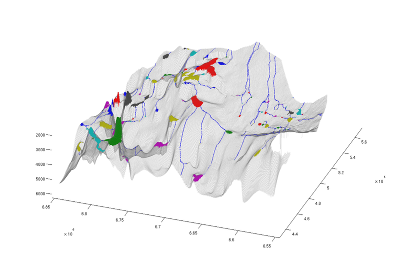#### Generate and visualize trapping information

This tutorial shows how to call the trapping analysis routine, and some ways to use the generated information.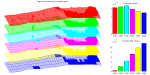#### Accuracy of structural trapping

When working with large datasets, accuracy is always limited, either due to limits on the dataset resolution or computational considerations. This example demonstrates and estimates the fine scale information that is lost, and how it relates to CO2 migration.

#### Top-surface grid

We show how to make a 2D top-surface grid suitable for vertical equilibrium simulations of a small 3D grid model. The tutorial introduces and explains the basic data structure used to represent the vertically integrated model in the VE solvers.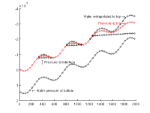#### Comparison of VE formulations

There are several ways to formulate a vertical equilibrium model. This example demonstrates and compares two approaches: One uses the height h of the CO2 column as the primary variable, the other the fractional height S=h/H.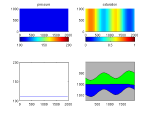#### VE in a regular black-oil solver

In this example we show how to set up a standard format black-oil model that can be used to simulate a VE model. For the actual simulation, we use the fully-implicit solver in MRST from the 'ad-fi' module, which is based on automatic differentiation.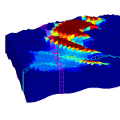#### Small sloping aquifer

We make a model of a syntetic sloping aquifer and demonstrate the use of the vertical equilibrium module for simulating injection and migration of CO2 on this model.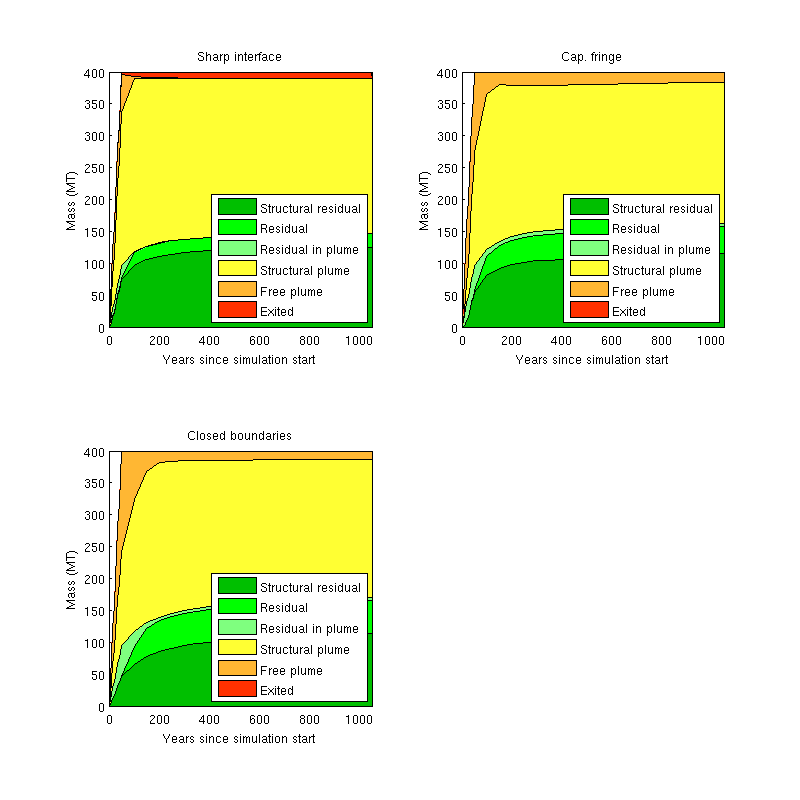#### Fully-implicit VE simulation using class-based black-oil programming framework

Since the MRST2015a, a new, class-based framework for setting up fully-implicit simulations in a simple way has been introduced.  Here, we use the framework to setup and run multiple simulations of an injection scenario, using different assumptions on boundary conditions and capillary pressure.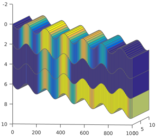#### Multilayered system with caprock leakage

This example shows a conceptual model of a multilayered system where there is leakage through the caprock that delineates each layer. The system is simulated using a standard fully-implicit simulator with a fluid model that mimics a vertical equilibrium formulation.

Published February 8, 2016